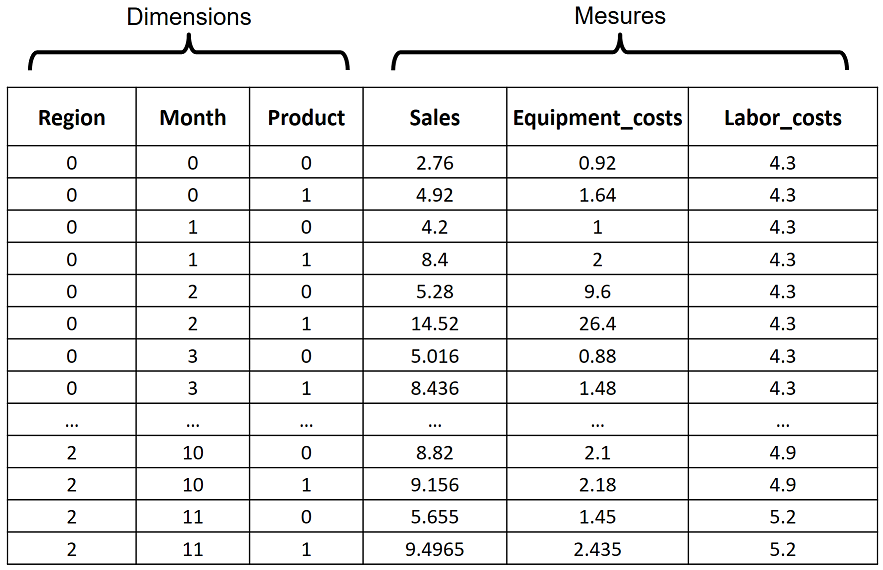# Barchart Matrix implemented with d3

Consider the following dataset stored as a table with 6 columns.The first 3 columns in the table are dimensions (independent, categorical variables), and the other 3 columns are measures (dependent, quantitative variables). The measures are functions of the dimensions. Each combination of the values of the dimensions occurs once. Since there are 3 Regions, 12 Months, and 2 Products, there are 3x12x2 = 72 rows.

The Barchart Matrix below provides an overview of the data in the table. The 3 dimensions are crossed with the 3 measures to produce a matrix, with each cell showing one measure as a function of one dimension.

(For d3 source code, view the source of this webpage.)

# Other Matrix-based Visualizations

Other visualizations that use a matrix of charts include